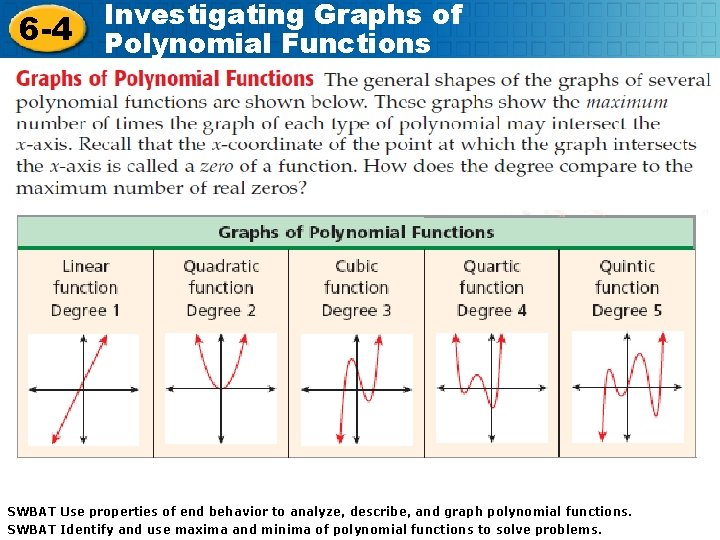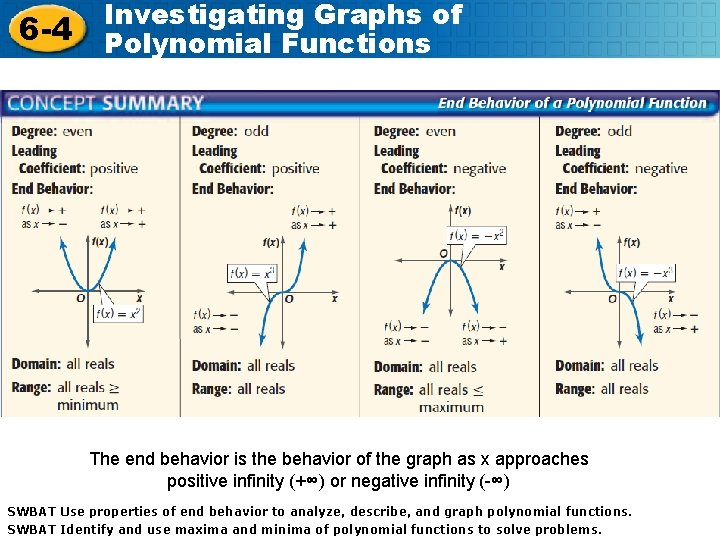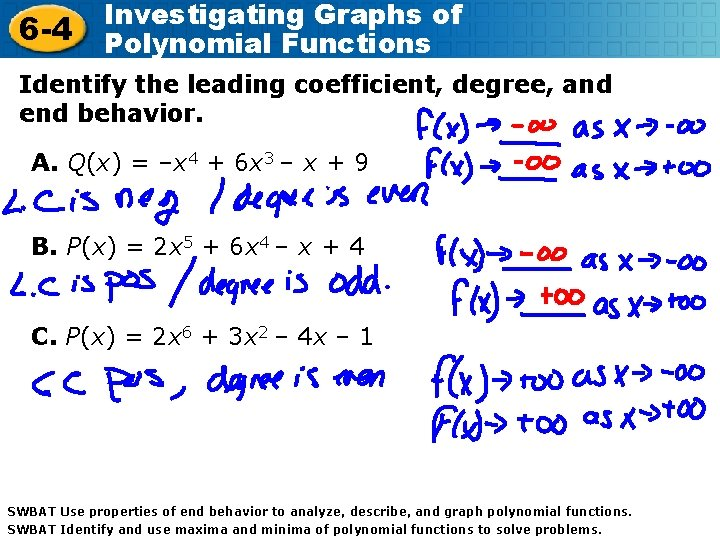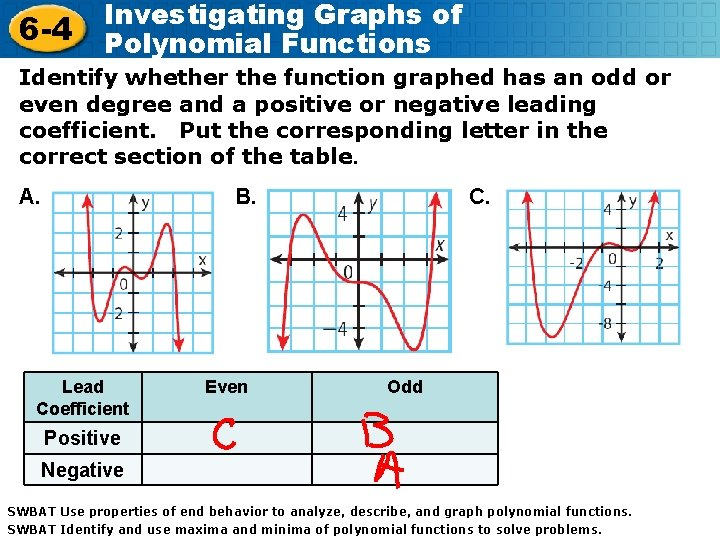# Investigating Graphs of 6 4 Polynomial Functions SWBAT

• Slides: 5
Download presentationInvestigating Graphs of 6 -4 Polynomial Functions SWBAT Use properties of end behavior to analyze, describe, and graph polynomial functions. SWBAT use maxima minima ofpolynomial functions SWBAT Use Identify properties ofand end behavior to analyze, and describe, and graph functions. Holt Mc. Dougal Algebra 2 Algebra SWBAT Identify and use maxima and minima of polynomial functions to solve problems. Holt Mc. Dougal 22 to solve problems.6 -4 Investigating Graphs of Polynomial Functions SWBAT Use properties of end behavior to analyze, describe, and graph polynomial functions. SWBAT Identify and use maxima and minima of polynomial functions to solve problems. Holt Mc. Dougal Algebra 26 -4 Investigating Graphs of Polynomial Functions The end behavior is the behavior of the graph as x approaches positive infinity (+∞) or negative infinity (-∞) SWBAT Use properties of end behavior to analyze, describe, and graph polynomial functions. SWBAT Identify and use maxima and minima of polynomial functions to solve problems. Holt Mc. Dougal Algebra 26 -4 Investigating Graphs of Polynomial Functions Identify the leading coefficient, degree, and end behavior. A. Q(x) = –x 4 + 6 x 3 – x + 9 B. P(x) = 2 x 5 + 6 x 4 – x + 4 C. P(x) = 2 x 6 + 3 x 2 – 4 x – 1 SWBAT Use properties of end behavior to analyze, describe, and graph polynomial functions. SWBAT Identify and use maxima and minima of polynomial functions to solve problems. Holt Mc. Dougal Algebra 26 -4 Investigating Graphs of Polynomial Functions Identify whether the function graphed has an odd or even degree and a positive or negative leading coefficient. Put the corresponding letter in the correct section of the table. A. B. Lead Coefficient Even C. Odd Positive Negative SWBAT Use properties of end behavior to analyze, describe, and graph polynomial functions. SWBAT Identify and use maxima and minima of polynomial functions to solve problems. Holt Mc. Dougal Algebra 2